Answer the questions on the basis of the inf...
Answer the questions on the basis of the information given below:
A, B, C. D and E are five brothers. They have assets in the form of flats and cash only. Together they have 9 flats and each brother has at least 1 flat. Value (in terms of rupees) of all flats is equal and constant. Cash with each brother is an integral multiple of Rs. 10 lakhs. The amount of cash with B is just enough to buy one more flat, all the other brothers have cashless than that of B. E has got the maximum number of flats. A number of flats with A is equal to the sum of the number of flats with B and C. Amount of cash with B is double of that with D, but D has got assets worth Rs. 20 lakhs more than that of B.
For how many brothers is it possible to find out the exact value of their total assets?
• a)
1
• b)
2
• c)
3
• d)
4
Answer the questions on the basis of the information given below:A, B...
E has got the maximum number of flats and number of flats with A is equal to the sum of a number of flats with B and C. As there are 9 flats, so possible solutions are when E, A, B, C and D have 3, 2, 1, 1 and 2 or 4, 2, 1. 1 and 1 flats respectively.
Case I:B and D have one flat and two flats respectively.
Let the value of a flat be Rs. x. So, the amount of cash with B = x.
So total assets of B = 2x
Total assets of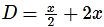As D has got assets worth 20 lakhs more than that of B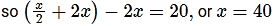Case II:
B and D have one flat each.
Let value of a flat be Rs. x. So, amount of cash with B = x
⇒ Total assets of B = 2x
Total assets of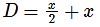As D has got assets worth 20 lakhs more than B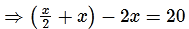Here the value of x is coming as negative. So this case is not possible.
We can collate the final distribution of assets in the following table: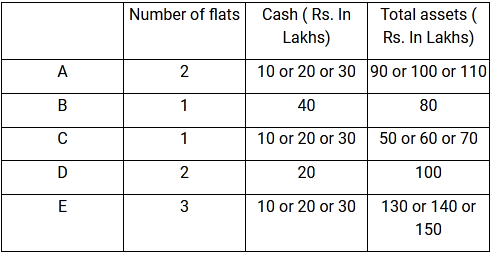According to the table B and D buy 1 and 2 flat respectively.
So, Only for B and D, it is possible to find the exact value of their total assets.​
Only 2 brothers find out the exact value of their total assets.
Hence the correct option is B.
 1 Crore+ students have signed up on EduRev. Have you?
1 Crore+ students have signed up on EduRev. Have you?

### Learn this topic in detailIPMAT Mock Test - 8 (New Pattern) 100 Ques | 90 MinsMore from Related Course Quantitative Aptitude (Quant)View courses related to this question

### Quick links for CAT exam850+
Video Lectures2500+
Revision Notes600+
Online Tests10,000+
Doubts Solved
Answer the questions on the basis of the information given below:A, B, C. D and E are five brothers. They have assets in the form of flats and cash only. Together they have 9 flats and each brother has at least 1 flat. Value (in terms of rupees) of all flats is equal and constant. Cash with each brother is an integral multiple of Rs. 10 lakhs. The amount of cash with B is just enough to buy one more flat, all the other brothers have cashless than that of B. E has got the maximum number of flats. A number of flats with A is equal to the sum of the number of flats with B and C. Amount of cash with B is double of that with D, but D has got assets worth Rs. 20 lakhs more than that of B.For how many brothers is it possible to find out the exact value of their total assets?a)1b)2c)3d)4Correct answer is option 'B'. Can you explain this answer?
Question Description(Scan QR code)